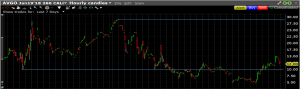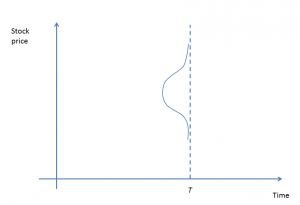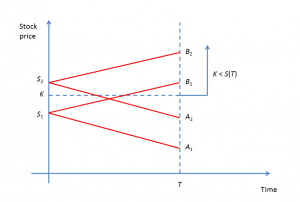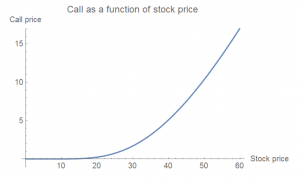25
Mar 18

18
Mar 18

## Intro to option greeks: theta, intrinsic value and time value

This world is full of surprises and uncertainties. One thing is for sure: time is unidirectional and nobody grows younger. This is true also for options: other things being equal, the value of the call option decreases with time. The dependence of option prices on time is the simplest but it allows us to discuss important related notions - the intrinsic value and time value.

## Exercising options

American options can be exercised before expiration. If you hold a long American call and it is in the money, you can request your broker that your option be exercised. That is, you buy the stock at the strike from the holder of the short call and gain the difference between the stock and strike prices. European options can be exercised only at expiration. Most of the time, exercising an option is not good because by acquiring stock you tie your money up in a larger investment and you lose what is called a time value.

For an ITM call, the difference "current stock price minus strike" is called its intrinsic value. This is how much you would profit if the call was exercised right away. For an OTM call, the intrinsic value is zero because you would not buy the stock at the price higher than the market price.Figure 1. Call option intrinsic value

14
Mar 18

## Intro to option greeks: delta and its determinants

I started trading stocks in 2010. I didn't expect to make big profits and wasn't actively trading. That's until 2015, when I met a guy who turned $10,000 into$140,000 in four years. And then I thought: why am I fooling around when it's possible to make good money? Experienced traders say: trading is a journey. That's how my journey started. Stocks move too slowly, to my taste, so I had to look for other avenues.

Two things were clear to me. I didn't want to be glued to the monitor the whole day and didn't want to study a lot of theory. Therefore I decided to concentrate on the futures market. To trade futures, you don't even need to know the definition of a futures contract. The price moves very quickly, and if you know what you are doing, you can make a couple of hundreds in a matter of minutes. It turned out that the futures markets are the best approximation to the efficient market hypothesis. Trend is your friend (until the end), as they say. In the futures markets, trends are rare and short-lived. Trading futures is like driving a race car. The psychological stress is enormous and it may excite your worst instincts. After trying for almost two years and losing $8,000 I gave up. Don't trade futures unless you can predict a big move. Many people start their trading careers in the forex market because the volumes there are large and transaction fees are low. I never traded forex and think that it is as risky as the futures market. If you want to try it, I would suggest to trade not the exchange rates themselves but indexes or ETF's (exchange traded funds) that trace them. Again, look for large movements. One more market I don't want to trade is bonds. Actions of central banks and macroeconomic events are among strong movers of this market. Otherwise, it's the same as futures. Futures, forex and bonds have one feature in common. In all of them institutional (large) traders dominate. My impression is that in absence of market-moving events they select a range within which to trade. Having deep pockets, they can buy at the top of the range and sell at the bottom without worrying about the associated loss. Trading in a range like that will kill a retail (small) investor. Changes in fundamentals force the big guys to shift the range, and that's when small investors have a chance to profit. I tried to avoid options because they require learning some theory. After a prolonged resistance, I started trading options and immediately fell in love with them. I think that anybody with$25,000 in savings can and should be trading options.

## Efficient market hypothesis

This hypothesis is a standard assumption in Economics. It states that market prices reflect "all relevant" information. The exact definition of the "relevant" information depends on how strong economists want the hypothesis to be. The main practical implication is that it's impossible to make profits in financial markets. I fail to logically connect the statement "market prices reflect all information" with the statement that "it's impossible to make profits". But my main point is not this.

The main point is that economists themselves indicate reasons why the efficient market hypothesis may not hold. One is the cost of obtaining information. The option chain for Apple for each expiration date contains dozens of strikes, and each strike means a separate market, although there is a high correlation between these markets. Multiply that number of markets by the number of expiration dates (weekly options give about 50 expiration dates and monthly options give another 12, that's for one year), and you will see that Apple alone generates hundreds, if not thousands, of markets.

Another reason why the hypothesis may not hold is transaction costs. The bid-ask spread (which is the difference ask-bid)  in the lower part of the above option chain is $7 and in the upper part is$50. In the options markets, the transaction costs and the cost of obtaining information will prevent any big player from attempting to capture all profits.

Dare, and you will be rewarded!

4
Mar 18

## Interest rate - the puppetmaster behind option pricesFigure 1. Call as a function of interest rate

The interest rate is the last variable we need to discuss. The dependence of the call price on the interest rate that emerges from the Black-Scholes formula is depicted in Figure 1. The dependence is positive, right? Not so fast. This is the case when common sense should be used instead of mathematical models. One economic factor can influence another through many channels, often leading to contradicting results.

John Hull offers two explanations.

1. As interest rates in the economy increase, the expected return required by investors from the stock tends to increase. This suggests a positive dependence of the call price on the interest rate.
2. On the other hand, when the interest rate rises, the present value of any future cash flow received by the long call holder decreases. In particular, this reduces the payoff if at expiration the option is in the money.

The combined impact of these two effects embedded in the Black-Scholes formula is to increase the value of the call options.

## However, experience tells the opposite

The Fed changes the interest rate at discrete times, not continually. Two moments matter: when the rumor about the upcoming interest rate change hits the market and when the actual change takes place. The market reaction to the rumor depends on the investors' mood - bullish or bearish. A bullish market tends to shrug off most bad news. In a bearish market, even a slight threat may have drastic consequences. By the time the actual change occurs, it is usually priced in.

In 2017, the Fed raised the rate three times: on March 15 (no reaction, judging by S&P 500 SPDR SPY), June 14 (again no reaction) and December 13 (a slight fall). The huge fall in the beginning of February 2018 was not caused by any actual change. It was an accumulated result of cautiousness ("This market has been bullish for too long!") and fears that the Fed would increase the rates in 2018 by more than had been anticipated. Many investors started selling stocks and buying bonds and other less risky assets. The total value of US bonds is about $20 trillion, while the total market capitalization of US stocks is around$30 trillion. The two markets are comparable in size, which means there is enough room to move from one to another and the total portfolio reshuffling can be considerable. Thus far, the mere expectation that the interest rate will increase has been able to substantially reduce stock prices and, consequently, call prices.

All this I summarized to my students as follows. When interest rates rise, bonds become more attractive. This is a substitution effect: investors switch from one asset to another all the time. Therefore stock prices and call prices fall. Thus the dependence of call prices on interest rates is negative.

The first explanation suggested by Hull neglects the substitution effect. The second explanation is not credible either, for the following reason. As I explained, stock volatility has a very strong influence on options. Options themselves have an even higher volatility. A change in interest rates by a couple percent is nothing in comparison with this volatility. Most investors would not care about the resulting reduction in the present value of future cash flows.

25
Feb 18

## Volatility - king among option price determinants

Here we apply the simple probabilistic model developed previously to see how a call price depends on volatility. Assumption 1 and the method to find ranges of initial stock prices that can lead to ITM areas give Figure 1.Figure 1. Dependence on volatility

As before, we are interested in the event$\{K that the call with the strike price$K$ is in the money at expiration. In Figure 1,$S_1$ corresponds to volatility$\sigma_1$ and$S_2$ corresponds to volatility$\sigma_2$, where$\sigma_1<\sigma_2$$S_1$ is the highest stock price such that its area of influence (the base of the corresponding rectangle) does not contribute to the ITM area. Hence, only stock prices higher than$S_1$ do (these prices are indicated by the green arrow). The same goes for$S_2$, the range of prices that contribute to the ITM area being shown by the blue arrow. We see that the area corresponding to the larger volatility is wider, and therefore the corresponding call is more expensive.Figure 2. Call as a function of volatility

In Figure 1, the independent variable (on the horizontal axis) is time. Figure 2 from Mathematica illustrates the same dependence with volatility as the independent variable.

## The Black-Scholes formula doesn't tell the full story

In the Black-Scholes formula, the volatility parameter is fixed. In practice, it changes all the time. The dependence on volatility, when the latter is very large, can dominate all other determinants. This is best illustrated with an example.Figure 3. AVGO stock

Figure 3 is the chart of AVGO (symbol of Broadcom Ltd) in the period of high volatility 10/30/2017-1/8/2018. In general, it's difficult to pick exactly tops and bottoms. But suppose you were able to do so buying the stock at $248 and selling at$286. Usually stocks are traded in batches of 100. In that case, your profit would be (286-248)*100=3800 and the return would be 3800/24800=15.3%. Let's compare this return with the one you could have trading calls.Figure 4. AVGO 260 call

In Figure 4 you can see the behavior of 260 call option that expired on January 19, 2018. The period is the same and both charts are hourly charts. One call option commands 100 shares of stock. Again, assuming that you were able to pick the bottom and top exactly, your profit would be (29-10)*100=1900 and the return would be 1900/1000=190%. You would have a much higher return with a much smaller investment!

Note also that in the subsequent period, when the volatility and stock price both fell, the stock price did not fall below the previous low of $248, while the option price broke the previous low of$10 and hit $5 at some point. Conclusion. The key to trading options is to catch a moment when volatility is low and is about to jump or when it is high and is going to fall. 23 Feb 18 ## Call options and probabilistic intuition - dependence on time ## Call options and probabilistic intuition - dependence on time As before, we change one variable - in this case it's time to expiration$T$, while holding others fixed, and see how the call price changes. We've assumed that at each$T$, the stock price distribution is triangular, symmetric and centered at$S(0)$. This time on top of that assumption we add the following. Assumption 2. The standard deviation of the stock price distribution is proportional to$T$. This standard deviation is a measure of riskiness and unpredictability of the stock price. The farther into the future, the more difficult it is to predict. ## Areas of influence of the initial stock priceFigure 1. Areas of influence for two times As a result of our assumptions, we can visualize areas of influence for two times in Figure 1. Points$S(0),\ A_1,\ A_2$ are on the same straight line. Similarly, points$S(0),\ B_1,\ B_2$ are on the same straight line. This is because by Assumption 2, the triangles$\triangle S(0),A_1,B_1$ and$\triangle S(0),A_2,B_2$ are similar. Note that the triangle$\triangle A_2, B_2,C_2$ is flatter than$\triangle A_1, B_1,C_1$ because in both cases the area should be 1. ## Finding ranges of initial stock prices that can lead to ITM areasFigure 2. Initial price ranges In Figure 2 we find initial price ranges that may contribute to the ITM areas. For example, for time$T_1$ we find the highest initial price$S_1$ such that its area of influence doesn't intersect the ITM area$\{K. Only initial prices above$S_1$ can contribute to$\{K (such prices are shown by the green arrow). Similarly, only initial prices above$S_2$ can contribute to$\{K (such prices are shown by the blue arrow). Since the blue area is wider than the green area, the call expiring at$T_2$ must be more expensive than the one expiring at$T_1$. ## Fast time decay of OTM optionFigure 3. Call price as a function of time to expiration If an OTM option continues to be OTM as expiration approaches, the likelihood of it becoming ITM quickly declines and the call price erodes to zero. This is illustrated in Figure 3 (from Mathematica), where time is measured in years, so the chart traces the call price as time to expiration declines from half a year to zero. The practical recommendation is to sell the option before it dies on you, if the odds of the stock moving higher are low. 20 Feb 18 ## Call options and probabilistic intuition - dependence on stock price ## Call options and probabilistic intuition - dependence on stock price The "Why do we want options?" section should be enough to understand that the call price grows when the stock price increases. Here we look at a more technical (linked to probabilities) explanation of that fact. ## Stock price as a stochastic process The stock price is a stochastic process. That is, it depends on two variables: time$T$ and element of the sample space$\omega$,$S=S(T,\omega)$.Figure 1. Stock price distribution If we fix time, we obtain a random variable whose density can be visualized as in Figure 1 (the element of the sample space is varying along the vertical line through$T$). The dependence on$\omega$ most of the time is not explicitly mentioned.Figure 2. Stock price If we fix the element of the sample space, we obtain a function of time, which is a particular path that the stock price may follow. It looks much like a Brownian motion path that I downloaded from Google images, except that the stock price cannot be negative, see Figure 2. ## Assumptions about stock behavior The distribution in Figure 1 looks like normal. Normal distributions are not good for modeling stock prices because stock prices cannot be negative or very large. In our analysis we use triangular distributions, which are isosceles triangles with area equal to one. Without any knowledge about the company and its stock, the best guess that we can make about the mean of the stock price$ES(T)$ at a future time is that it is equal to the stock price now$S(0)$. Assumption 1. The distribution of$S(T)$ is triangular and it is centered at$S(0)$.Figure 3. Triangular distribution The result of this assumption is Figure 3, where the triangle ABC describes the density of$S(T)$. We also need the triangle S(0)AB, which describes the area of influence of the price$S(0)$: stock price paths that start at$S(0)$ may end only in the base AB of the triangle. Note that if the price$S(0)$ is low, then the triangle S(0)AB intersects the time line and negative prices will be possible. To avoid complications, we exclude low stock prices. ## Influence of stock price on call priceFigure 4. Call price as a function of stock price In Figure 4 we compare two initial stock prices:$S_1. Recall that the call price is higher if the likelihood of the event$\{K is higher. For$S_1$ the influence area is the segment$A_1B_1$, which has a smaller intersection with$\{K than the segment$A_2B_2$, which is the influence area of$S_2$. Thus, the call price corresponding to$S_2$ should be higher than the one corresponding to$S_1$.Figure 5. Call as a function of stock price Figure 5 from Mathematica concludes this post. In that Figure, the strike price is assumed to be$50. The very low call price at stock prices below $20 is due to the fact that it is not very likely for the stock to become in the money if it starts that low. 19 Feb 18 ## Call options and probabilistic intuition - dependence on strike ## Call options and probabilistic intuition - dependence on strike Awhile ago I gave a course in options following the excellent book by Hull. Frankly, at that time I didn't understand a fraction of what a trader needs to understand about options. I plan to make several posts to explain the intuition behind the Black-Scholes formula for options valuation. The geniality of this formula consists in the fact that it correctly reflects the likelihood of various events. More precisely, the more likely a certain event, the higher the call option price. The level of explanation will be midway between the theoretical view of Hull and practical view of option traders. We start with a series of definitions. ## Why do we want options? A stock price moves up and down, and this movement is pretty erratic. You don’t want to buy the stock, because it’s an expensive investment and the price may fall right after you buy the stock. However, in case the price goes up, you want to gain from that movement, without the financial commitment required by the stock purchase. You are willing to pay a relatively small amount for the guarantee that if the stock goes up, you will be able to buy it at a fixed (presumably, lower) price. At the same time, you don’t want to buy the stock if it goes down. This is the idea behind the call option. ## Basic definitions A call option gives its buyer the right, but not the obligation, to buy the stock at the price fixed in the option contract. This price is called a strike price and denoted$K$. The contract is valid until a certain date called an expiration date and denoted$T$. More generally,$T$ may denote any time before expiration and$S(T)$ will then denote the stock price at that time (it is random and depends on time, while the strike price is fixed for the life of the contract and is deterministic). There are two parties in the transaction: the call buyer owns a long call and the call seller owns a short call. We discuss only long positions; for short positions most of the time the opposite is true. At any point in time during the life of the option, the strike price$K$ may be higher than the stock price. In this case people say that it is out of the money (OTM). If$K=S$, the option is at the money (ATM). If$K, the option is in the money (ITM). The call buyer wants the call to be ITM at expiration. If at expiration the call option is ITM, the long call holder buys the stock at price$K$ and sells at$S(T)$, profiting$S(T)-K-c$, where$c$ is the call price paid at the outset. If at expiration the call option is ATM or OTM, the holder doesn't gain from buying the stock and gives up the right to buy it, because buying is not an obligation. The option expires worthless and the call holder’s loss is$c$. The market price$c$ of a call option depends on 5 variables: the strike price$K$, stock price$S$, time to expiration$T$ (measured in years), volatility of the stock price$\sigma$ and risk-free interest rate$r$ (annualized). Our purpose is to understand the option price dynamics when one of the arguments of the call option changes, while others are kept fixed. The call price changes during the life of the option, depending on market conditions. The call holder can sell it at any time prior to expiration. The call price at that time depends on the event$\{S(T)>K\}$ that the option is in the money. In all our explanations we will use this event. The higher its likelihood, the higher the call price. ## So, how does the call price depend on the strike price?Figure 1. In the money ranges for two strikes Consider two strike prices$K_1. Since$\{K_2 (see Figure 1), the$K_1$ call should be more expensive than the$K_2$ call because the market values higher events with higher probabilities. As the strike increases, the call price falls. This is illustrated in Figure 2.Figure 2. Call as a function of strike price Figure 2 was produced in Mathematica using the Black-Scholes formula, which is valid for European options that can be exercised only at expiration. Our simple probabilistic argument is true also for American options that can be exercised any time prior to expiration. In Figure 2 we assume that the stock price is$50 (the other assumptions are Expiration=0.5, Interest Rate=0.1, Volatility=0.5, Dividend=0.05). The red part of the curve corresponds to in the money strikes, and the out of the money strikes are shown by the blue part of the curve.

Note also that in Mathematica it's better to use the command FinancialDerivative than to program the Black-Scholes formula directly. The latter for some reason sometimes doesn't work.